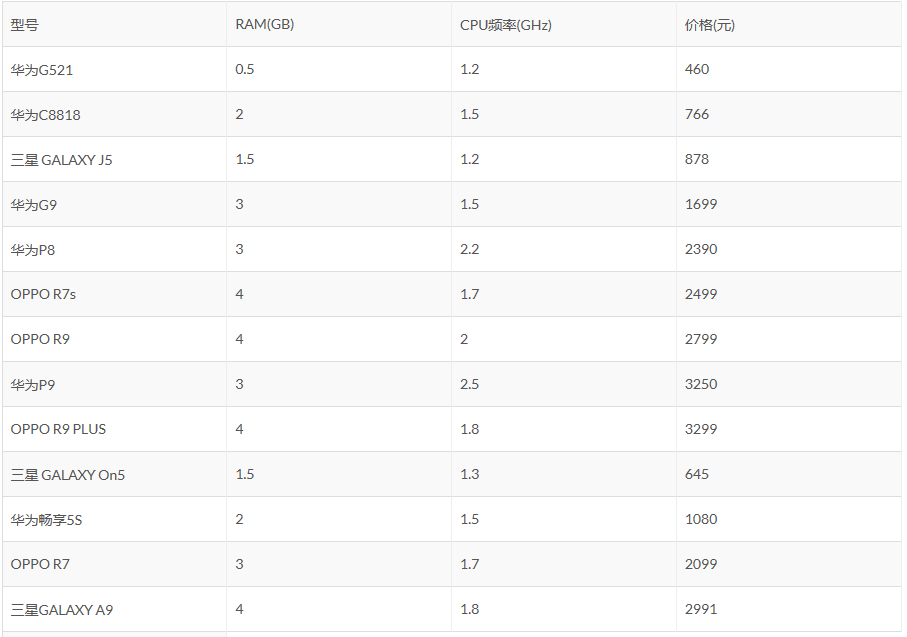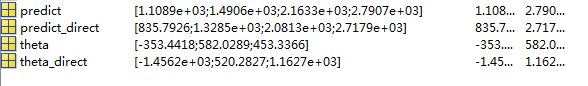# 回顾一下上篇使用的例子# 再谈批梯度下降

$$J(\theta)=\frac{1}{2}\sum_{i=1}^{m}(h(x^{(i)})-y^{(i)})^2$$

$$\frac{\partial}{\partial\theta_j}J(\theta)=\sum_{i=1}^m(h_\theta(x^{(i)})-y^{(i)})x_j^{(i)}$$

# 随机梯度下降1 公式求解

2 批梯度下降

3 随机梯度下降

# 参考资料

1 斯坦福机器学习公开课及其课程笔记 by Andrew Ng